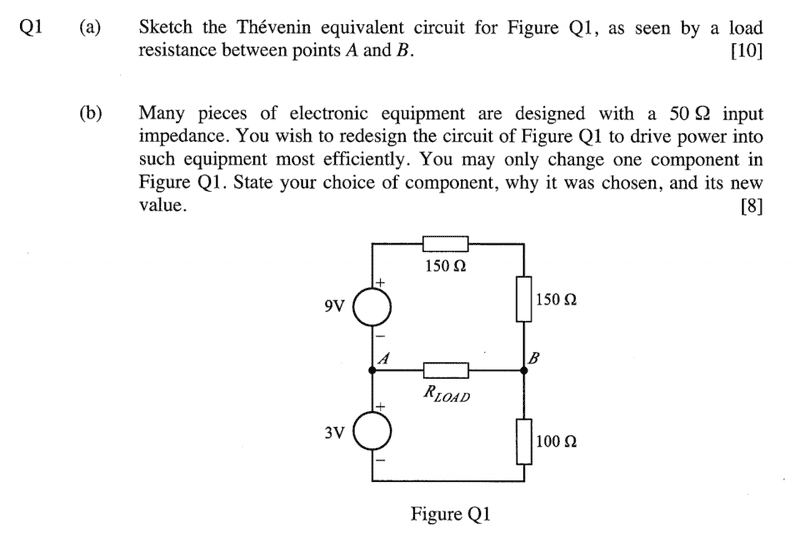# Thevenin Equivalent Circuit

• Engineering

## Homework Statement

See attached image## Homework Equations

Thevenin Equivalent

## The Attempt at a Solution

a) Find the total resistance between A and B by short circuiting the voltage sources:

Rt = 100Ω in parallel with the two 150Ω

Two 150Ω in series: R = 300Ω
1/Rt = 1/100 + 1/300

Rt = 75Ω

I am having difficulty finding the thevenin equivalent voltage.

b) Don't entirely understand the question so I am unable to make an attempt

Any help would be greatly appreciated.
Thanks

gneill
Mentor
I am having difficulty finding the thevenin equivalent voltage.
What have you tried? What's the usual procedure?
b) Don't entirely understand the question so I am unable to make an attempt
Hint: Maximum power transfer theorem.

•Ash_M
What have you tried? What's the usual procedure?

Hint: Maximum power transfer theorem.

I have no idea so I have not tried anything. Usually for a system with a single voltage source I would try to find the open circuit voltage between A and B, however the two voltage sources are confusing me.

Oh so would I take the Rload to be 50Ω, and since for maximum power the thevenin resistance = load resistance, change one of the resistors to give me a thevenin resistance of 50Ω?

gneill
Mentor
I have no idea so I have not tried anything. Usually for a system with a single voltage source I would try to find the open circuit voltage between A and B, however the two voltage sources are confusing me.
You can always do a "KVL walk" from A to B and sum up the potential changes along the way.
Oh so would I take the Rload to be 50Ω, and since for maximum power the thevenin resistance = load resistance, change one of the resistors to give me a thevenin resistance of 50Ω?
Yes.

•Ash_M
You can always do a "KVL walk" from A to B and sum up the potential changes along the way.

Yes.

I don't quite understand what you mean by a "KVL walk", but i tried going round the top of the circuit and noting how the potential would change I also wasn't sure if the current in the entire system could be calculated as I = 12/400 . Doing that would mean I get a voltage of 0V at A and then 0V at B which can't be correct.

gneill
Mentor
I don't quite understand what you mean by a "KVL walk", but i tried going round the top of the circuit and noting how the potential would change I also wasn't sure if the current in the entire system could be calculated as I = 12/400 . Doing that would mean I get a voltage of 0V at A and then 0V at B which can't be correct.You did well, and a "KVL walk" is just what you did.

0 V is a perfectly valid result.

•Ash_MYou did well, and a "KVL walk" is just what you did.

0 V is a perfectly valid result.
Haha thanks so much!You did well, and a "KVL walk" is just what you did.

0 V is a perfectly valid result.

I took a different approach to this question, calculating Vb from 0 = (Vb-9)/300 + (Vb-(-3))/100, so Vb = 0. Is there a way to get a nodal equation for Va?

gneill
Mentor
I took a different approach to this question, calculating Vb from 0 = (Vb-9)/300 + (Vb-(-3))/100, so Vb = 0. Is there a way to get a nodal equation for Va?
You've used A as the reference node for your first equation, so it's potential is defined to be zero. That is, Va = 0 is the node equation for AYou've used A as the reference node for your first equation, so it's potential is defined to be zero. That is, Va = 0 is the node equation for AWhat do you mean by reference node?

cnh1995
Homework Helper
Gold Member
What do you mean by reference node?
A node whose potential is taken as 0V for reference. All the voltages in the circuit are measured with respect to the reference node. It is also called as the "circuit ground".

gneill
Mentor
What do you mean by reference node?
When you do nodal analysis you are determining the potentials of various nodes of the circuit with respect to some reference node. The first step is always to choose the node that will be the reference node for all the potentials.

There's often an obvious or convenient node to choose for this role (what constitutes "obvious" or "convenient" and how to recognize a good choice for the reference node is something that comes with experience). In this case node A is suitable since it not only forms one of the "output" terminals of the Thevenin equivalent, but is located at the junction of the only two voltage sources in the circuit.

A node whose potential is taken as 0V for reference. All the voltages in the circuit are measured with respect to the reference node. It is also called as the "circuit ground".

When you do nodal analysis you are determining the potentials of various nodes of the circuit with respect to some reference node. The first step is always to choose the node that will be the reference node for all the potentials.

There's often an obvious or convenient node to choose for this role (what constitutes "obvious" or "convenient" and how to recognize a good choice for the reference node is something that comes with experience). In this case node A is suitable since it not only forms one of the "output" terminals of the Thevenin equivalent, but is located at the junction of the only two voltage sources in the circuit.

Ok. Cool. Thanks Guys. Any time we did a problem in lectures, the ground was always marked in, so I never realised you could 'pick' a ground.